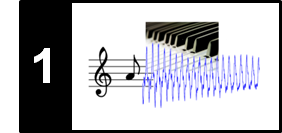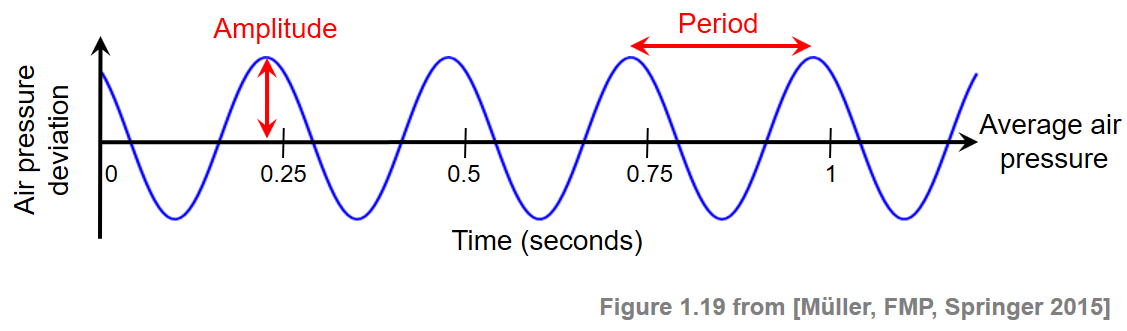# Frequency and Pitch

Following Section 1.3.2 of [Müller, FMP, Springer 2015], we cover in this notebook the relation between frequency and pitch.

## Sinusoids¶

A sound wave can be visually represented by a waveform. If the points of high and low air pressure repeat in an alternating and regular fashion, the resulting waveform is called periodic. In this case, the period of the wave is defined as the time required to complete a cycle. The frequency, measured in Hertz (Hz), is the reciprocal of the period. The simplest type of periodic waveform is a sinusoid, which is completely specified by its frequency, its amplitude (the peak deviation of the sinusoid from its mean), and its phase (determining where in its cycle the sinusoid is at time zero). The following figure shows a sinusoid with frequency $4~\mathrm{Hz}$.## Audible Frequency Range¶

The higher the frequency of a sinusoidal wave, the higher it sounds. The audible frequency range for humans is between about $20~\mathrm{Hz}$ and $20000~\mathrm{Hz}$ ($20~\mathrm{kHz}$). Other species have different hearing ranges. For example, the top end of a dog's hearing range is about $45~\mathrm{kHz}$, a cat's is $64~\mathrm{kHz}$, while bats can even detect frequencies beyond $100~\mathrm{kHz}$. This is why one can use a dog whistle, which emits ultrasonic sound beyond the human hearing capability, to train and to command animals without disturbing nearby people.

In the following experiment, we generate a chirp signal which frequency increases by a factor of two (one octave) every second. Starting with $80~\mathrm{Hz}$, the frequency raises to $20480~\mathrm{Hz}$ over a total duration of $8$ seconds.

In :
import IPython.display as ipd
import numpy as np
import sys

sys.path.append('..')
import libfmp.c1

Fs = 44100
dur = 1
freq_start = 80 * 2**np.arange(8)
for f in freq_start:
if f==freq_start:
chirp, t = libfmp.c1.generate_chirp_exp_octave(freq_start=f, dur=dur, Fs=Fs, amp=1)
else:
chirp_oct, t = libfmp.c1.generate_chirp_exp_octave(freq_start=f, dur=dur, Fs=Fs, amp=1)
chirp = np.concatenate((chirp, chirp_oct))

ipd.display(ipd.Audio(chirp, rate=Fs))